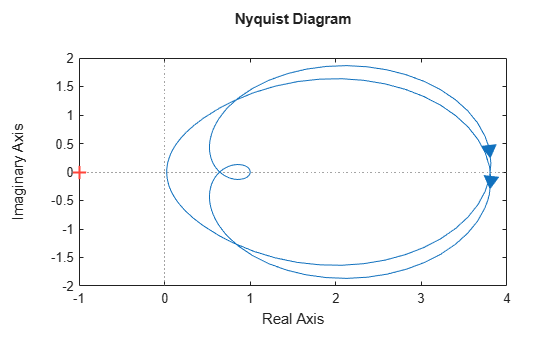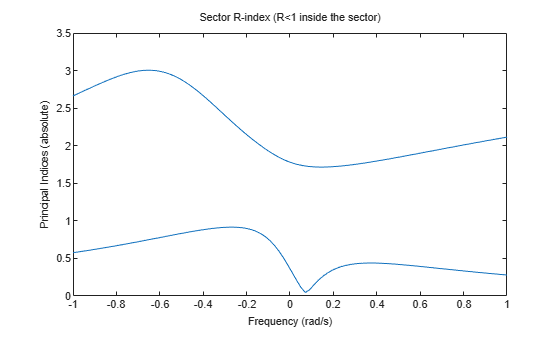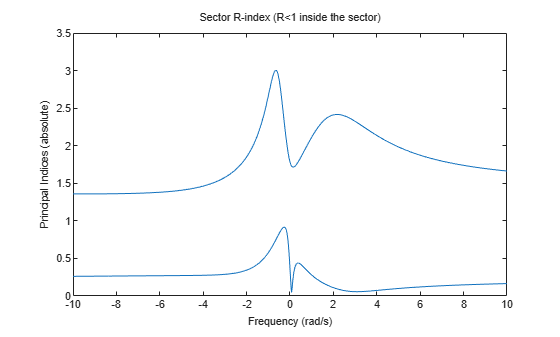getPassiveIndex

Compute passivity index of linear system

Description

getPassiveIndex computes various measures of the excess or shortage of passivity for a given system.

A linear system G(s) is passive if all its I/O trajectories (u(t),y(t)) satisfy:

${\int }_{0}^{T}y{\left(t\right)}^{T}u\left(t\right)dt>0,$

for all T > 0. Equivalently, a system is passive if its frequency response is positive real, such that for all ω > 0,

$G\left(j\omega \right)+G{\left(j\omega \right)}^{H}>0$

(or the discrete-time equivalent).

example

R = getPassiveIndex(G) computes the relative passivity index. G is passive when R is less than one. R measures the relative excess (R < 1) or shortage (R > 1) of passivity.

example

nu = getPassiveIndex(G,'input') computes the input passivity index. The system is input strictly passive when nu > 0. nu is also called the input feedforward passivity (IFP) index. The value of nu is the minimum feedforward action such that the resulting system is passive.

example

rho = getPassiveIndex(G,'output') computes the output passivity index. The system is output strictly passive when rho > 0. rho is also called the output feedback passivity (OFP) index. The value of rho is the minimum feedback action such that the resulting system is passive.

example

tau = getPassiveIndex(G,'io') computes the combined I/O passivity index. The system is very strictly passive when tau > 0.

DX = getPassiveIndex(G,dQ) computes the directional passivity index in the direction specified by the matrix dQ.

index = getPassiveIndex(___,tol) computes the passivity index with relative accuracy specified by tol. Use this syntax with any of the previous combinations of input arguments. index is the corresponding passivity index R, nu, rho, tau, or DX.

index = getPassiveIndex(___,tol,fband) computes passivity indices restricted to a specified frequency interval.

example

[index,FI] = getPassiveIndex(___) also returns the frequency at which the returned index value is achieved.

[index,FI,Qout,dQout] = getPassiveIndex(___) also returns the sector matrix Qout for passivity and the directional index matrix dQout.

Examples

collapse all

Compute passivity indices for the following dynamic system:

$G\left(s\right)=\frac{{s}^{2}+s+5s+0.1}{{s}^{3}+2{s}^{2}+3s+4}$

G = tf([1,1,5,.1],[1,2,3,4]);

Compute the relative passivity index.

R = getPassiveIndex(G)
R = 0.9512

The system is passive, but with a relatively small excess of passivity.

Compute the input and output passivity indices.

nu = getPassiveIndex(G,'input')
nu = 0.0250
rho = getPassiveIndex(G,'output')
rho = 0.2583

This system is both input strictly passive and output strictly passive.

Compute the combined I/O passivity index.

tau = getPassiveIndex(G,'io')
tau = 0.0250

The system is very strictly passive as well. A system that is very strictly passive is also strictly positive real. Examining the Nyquist plot confirms this, showing that the frequency response lies entirely within the right half-plane.

nyquistplot(G)The relatively small tau value is reflected in how close the frequency response comes to the imaginary axis.

For systems with complex coefficients, getPassiveIndex can return indices at a negative or positive frequency depending on the fband you specify.

Load the state-space model with complex data.

Compute the relative passivity index and its frequency with a relative accuracy of 0.0001%. Also, specify fband = [0.1,1] to compute the index in the frequency interval [–1,–0.1] ∪ [0.1,1].

[R,FI] = getPassiveIndex(sys,1e-6,[0.1,1])
R = 3.0050
FI = -0.6518

In this interval, sys achieves a relative passivity index of 3.0050 at a negative frequency value of –0.6518 rad/s. Use passiveplot to plot the indices in this range.

opt = sectorplotoptions;
opt.FreqScale = 'Linear';
opt.IndexScale = 'Linear';
w = linspace(-1,1,100);
passiveplot(sys,w,opt)Now compute the relative passivity index in the frequency interval [–10,–1.5] ∪ [1.5,10]. To do so, specify fband = [1.5,10].

[R,FI] = getPassiveIndex(sys,1e-6,[1.5,10])
R = 2.4162
FI = 2.1707

In this interval, sys achieves a relative passivity index of 2.4162 at a positive frequency value of 2.1707 rad/s. Plot the indices in this range to confirm the result.

w = linspace(-10,10,1000);
passiveplot(sys,w,opt)Input Arguments

collapse all

Model to analyze for passivity, specified as a dynamic system model such as a tf, ss, or genss model. G can be MIMO, if the number of inputs equals the number of outputs. G can be continuous or discrete. If G is a generalized model with tunable or uncertain blocks, getPassiveIndex evaluates passivity of the current, nominal value of G.

If G is a model array, then getPassiveIndex returns the passivity index as an array of the same size, where:

index(k) = getPassivityIndex(G(:,:,k),___)

Here, index is any of R, nu, rho, tau, or DX, depending on which input arguments you use.

Custom direction in which to compute passivity, specified as a symmetric square matrix that is 2*ny on a side, where ny is the number of outputs of G.

The rho, nu, and tau indices each correspond to a particular direction in the y/u space of the system, with a corresponding dQ value. (See dQout for these values.) Use this argument to specify your own value for this direction.

Relative accuracy for the calculated passivity index. By default, the tolerance is 1%, meaning that the returned passivity index is within 1% of the actual passivity index.

Frequency interval for determining passivity index, specified as an array of the form [fmin,fmax] with 0 ≤ fmin < fmax. When you provide fband, then getPassiveIndex restricts the frequency-domain computation of the passivity index to that frequency range. For example, the relative passivity index R is the peak gain of the bilinear-transformed system (I-G)(I+G)^-1 (for minimum-phase (I + G)). When you provide fband, then R is the peak gain within the frequency band.

For models with complex coefficients, getPassiveIndex computes the passivity index in the range [–fmax,–fmin][fmin,fmax]. As a result, the function can return indices at a negative frequency.

Specify frequencies in units of rad/TimeUnit, where TimeUnit is the TimeUnit property of the dynamic system model G.

Output Arguments

collapse all

Relative passivity index, returned as a scalar, or an array if G is an array.

The system G is passive when R is less than one.

• R < 1 indicates a relative excess of passivity.

• R > 1 indicates a relative shortage of passivity.

When I + G is minimum phase, R is the peak gain of the bilinear-transformed system (I - G)(I + G)^-1.

Input passivity index, returned as a scalar, or an array if G is an array. nu is defined as the largest value of ν for which:

${\int }_{0}^{T}y{\left(t\right)}^{T}u\left(t\right)dt>\nu {\int }_{0}^{T}u{\left(t\right)}^{T}u\left(t\right)dt,$

for all T > 0. Equivalently, nu is the largest ν for which:

$G\left(j\omega \right)+G{\left(j\omega \right)}^{H}>2\nu I$

(or the discrete-time equivalent). The system is input strictly passive when nu > 0. nu is also called the input feedforward passivity (IFP) index. The value of nu is the minimum feedforward action such that the resulting system is passive.

Output passivity index, returned as a scalar, or an array if G is an array. rho is defined as the largest value of ρ for which:

${\int }_{0}^{T}y{\left(t\right)}^{T}u\left(t\right)dt>\rho {\int }_{0}^{T}y{\left(t\right)}^{T}y\left(t\right)dt,$

for all T > 0. The system is output strictly passive when rho > 0. rho is also called the output feedback passivity (OFP) index. The value of rho is the minimum feedback action such that the resulting system is passive.

Combined I/O passivity index, returned as a scalar, or an array if G is an array. tau is defined as the largest value of τ for which:

${\int }_{0}^{T}y{\left(t\right)}^{T}u\left(t\right)dt>\tau {\int }_{0}^{T}\left(u{\left(t\right)}^{T}u\left(t\right)+y{\left(t\right)}^{T}y\left(t\right)\right)dt,$

for all T > 0. The system is very strictly passive when tau > 0.

Directional passivity index in the direction specified by dQ, returned as a scalar, or an array if G is an array. The directional passivity index is the largest value of D for which:

${\int }_{0}^{T}y{\left(t\right)}^{T}u\left(t\right)dt>D{\int }_{0}^{T}\left({\left(\begin{array}{c}y\left(t\right)\\ u\left(t\right)\end{array}\right)}^{T}dQ\left(\begin{array}{c}y\left(t\right)\\ u\left(t\right)\end{array}\right)\right)dt,$

for all T > 0. The rho, nu, and tau indices correspond to particular choices of dQ (see the output argument dQout). To compute DX, the software uses the custom dQ value you supply, dQ.

Frequency at which the returned passivity index is achieved, returned as a scalar, or an array if G is an array. In general, the passivity indices vary with frequency (see passiveplot). For each index type, the returned value is the largest value over all frequencies. FI is the frequency at which this value occurs, returned in units of rad/TimeUnit, where TimeUnit is the TimeUnit property of G.

FI can be negative for systems with complex coefficients.

Sector geometry used for computing the passivity index, returned as a matrix. For passivity indices, Qout is given by:

Qout = [zeros(ny),-1/2*eye(ny);-1/2*eye(ny),zeros(ny)];

where ny is the number of outputs of G. For example, for a SISO G,

Qout = [ 0,  -0.5;
-0.5, 0   ];

Direction in which passivity is computed, returned as a square matrix that is 2*ny on a side, where ny is the number of outputs of G. The value returned for dQout depends on what kind of passivity index you calculate:

• nu — For the input passivity index, dQout is given by:

dQout = [zeros(ny),zeros(ny);zeros(ny),eye(ny)];

For instance, for a SISO system, dQout = [0,0;0,1].

• rho — For the output passivity index, dQout is given by:

dQout = [eye(ny),zeros(ny);zeros(ny),zeros(ny)];

For instance, for a SISO system, dQout = [1,0;0,0].

• tau — For the combined I/O passivity index, dQout is given by:

dQout = eye(2*ny);

For instance, for a SISO system, dQout = [1,0;0,1].

• DXdQout is the custom value you provide in the dQ input argument.

• R — The relative passivity index does not involve a direction, so in this case the function returns dQout = [].